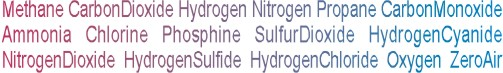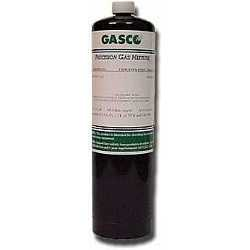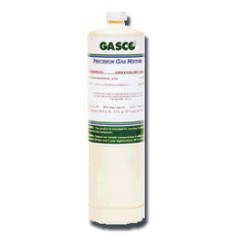Certified precision calibration gas mixtures and accessories for industrial gas leak detectors
NON-REACTIVE SINGLE GAS > OXYGEN :    41 Items found.(1515)

## NORLAB Equivalent  P107219

Norlab Equivalent Calibration Gas 19.0% Oxygen Balance nitrogen 17L Liter cylinder (Made by Gasco)

Configure

\$32.00(1506)

## NORLAB Equivalent  P107204

Norlab Equivalent Calibration Gas 0.4% Oxygen Balance nitrogen 17L Liter cylinder (Made by Gasco)

Configure

\$32.00(1516)

## NORLAB Equivalent  P1072195

Norlab Equivalent Calibration Gas 19.5% Oxygen Balance nitrogen 17L Liter cylinder (Made by Gasco)

Configure

\$32.00(1514)

## NORLAB Equivalent  P107218

Norlab Equivalent Calibration Gas 18.0% Oxygen Balance nitrogen 17L Liter cylinder (Made by Gasco)

Configure

\$32.00(1513)

## NORLAB Equivalent  P107217

Norlab Equivalent Calibration Gas 17.0% Oxygen Balance nitrogen 17L Liter cylinder (Made by Gasco)

Configure

\$32.00(1512)

## NORLAB Equivalent  P107215

Norlab Equivalent Calibration Gas 15.0% Oxygen Balance nitrogen 17L Liter cylinder (Made by Gasco)

Configure

\$32.00(1511)

## NORLAB Equivalent  P10728

Norlab Equivalent Calibration Gas 8.0% Oxygen Balance nitrogen 17L Liter cylinder (Made by Gasco)

Configure

\$32.00(1510)

## NORLAB Equivalent  P10727

Norlab Equivalent Calibration Gas 7.0% Oxygen Balance nitrogen 17L Liter cylinder (Made by Gasco)

Configure

\$32.00(1509)

## NORLAB Equivalent  P10725

Norlab Equivalent Calibration Gas 5.0% Oxygen Balance nitrogen 17L Liter cylinder (Made by Gasco)

Configure

\$32.00(1508)

## NORLAB Equivalent  P10724

Norlab Equivalent Calibration Gas 4.0% Oxygen Balance nitrogen 17L Liter cylinder (Made by Gasco)

Configure

\$32.00(1507)

## NORLAB Equivalent  P10722

Norlab Equivalent Calibration Gas 2.0% Oxygen Balance nitrogen 17L Liter cylinder (Made by Gasco)

Configure

\$32.00(1517)

## NORLAB Equivalent  P1072209

Norlab Equivalent Calibration Gas 20.9% Oxygen Balance nitrogen 17L Liter cylinder (Made by Gasco)

Configure

\$32.00(1527)

## NORLAB Equivalent  HP107219

Norlab Equivalent Calibration Gas 19.0% Oxygen Balance nitrogen 34LS Liter cylinder (Made by Gasco)

Configure

\$65.00(1518)

## NORLAB Equivalent  HP107204

Norlab Equivalent Calibration Gas 0.4% Oxygen Balance nitrogen 34LS Liter cylinder (Made by Gasco)

Configure

\$65.00(1519)

## NORLAB Equivalent  HP10722

Norlab Equivalent Calibration Gas 2.0% Oxygen Balance nitrogen 34LS Liter cylinder (Made by Gasco)

Configure

\$65.00(1520)

## NORLAB Equivalent  HP10724

Norlab Equivalent Calibration Gas 4.0% Oxygen Balance nitrogen 34LS Liter cylinder (Made by Gasco)

Configure

\$65.00(1521)

## NORLAB Equivalent  HP10725

Norlab Equivalent Calibration Gas 5.0% Oxygen Balance nitrogen 34LS Liter cylinder (Made by Gasco)

Configure

\$65.00(1522)

## NORLAB Equivalent  HP10727

Norlab Equivalent Calibration Gas 7.0% Oxygen Balance nitrogen 34LS Liter cylinder (Made by Gasco)

Configure

\$65.00(1523)

## NORLAB Equivalent  HP10728

Norlab Equivalent Calibration Gas 8.0% Oxygen Balance nitrogen 34LS Liter cylinder (Made by Gasco)

Configure

\$65.00(1524)

## NORLAB Equivalent  HP107215

Norlab Equivalent Calibration Gas 15.0% Oxygen Balance nitrogen 34LS Liter cylinder (Made by Gasco)

Configure

\$65.00
Page: *1 | 2 | 3 | All